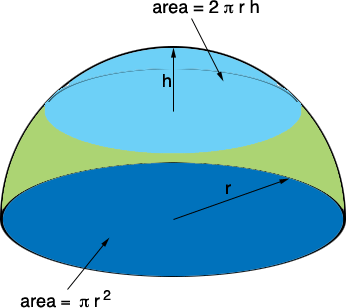SEARCH HOMEMath Central Quandaries & QueriesQuestion from Charlie: Why is the calculation for the surface area of a domed tank less than the surface area of a flat top tank. Using domed tank formula 2*pi*r(r-(r-dome ht) works out to be less surface area than a flat top tank area formula pi*r x r, Shouldn't the dome have more surface area than the flat surface ?Hi Charlie,

The expression you have for the surface area of a dome is correct (except you misses a parenthisis). It is

2 × π r(r - (r - h)) square units

where h is the dome height. This simplifies to

2 × π r × h square units.If, for example, h = r so that the dome (light blue) is half of the sphere then the surface area of the dome is 2 π r2 square units, twice the area ofthe base (dark blue). If h is very small then the area of the cap can be much smaller than the area of the base.

I hope this helps,
HarleyMath Central is supported by the University of Regina and The Pacific Institute for the Mathematical Sciences.